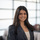# [Solution] Bucketing Values in Bins of 10

## Click here to view the question

Solution:

```select
(floor(my_value / 10.0) * 10 )::varchar(3) || ' to ' || (ceil(my_value / 10.0) * 10 )::varchar(3) as bucket
, count(*) as frequency
from
numbers
group by
1, (floor(my_value / 10.0) * 10 )
order by
(floor(my_value / 10.0) * 10 )```

Concepts Covered: Floor and Ceiling functions, Aggregations, Casting Data Types, Concatenation (Some may opt to use a Case When statement)

Explanation:

Note: since this table was generated using the Random() function, your exact frequencies may differ from the “Desired Output.”

One approach would be to create a case when in the select statement that tests to see if a value is under 10, then assign a value ‘0 to 10’, else if the value is under 20, assign a value ‘10 to 20,’ and so on. However, we can do this much more simply using floor and ceiling functions. In the solution above, we use the floor function to find the lower bound of the bucket, and the ceil function to find the upper bound. We then cast the results of the floor and ceiling as a value that can be concatenated with a string ‘ to ‘. Note that Redshift and PostgresSQL have a shortcut to cast data types (::). Other flavors of SQL can use a Cast function.

To get the frequency, we run a count(*), grouping by the first column. Note that we also need to group by the same field mentioned in the order by for this query to run in Redshift.